JMSLTM Numerical Library 7.2.0
com.imsl.math

## Class FeynmanKac

• All Implemented Interfaces:
Serializable, Cloneable

```public class FeynmanKac
extends Object
implements Serializable, Cloneable```
Solves the generalized Feynman-Kac PDE.

Class `FeynmanKac` solves the generalized Feynman-Kac PDE on a rectangular grid with boundary conditions using a finite element Galerkin method. The generalized Feynman-Kac differential equation has the form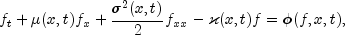where the initial data satisfies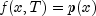. The derivatives are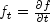, etc. Method `computeCoefficients` uses a finite element Galerkin method over the rectangle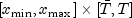in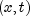to compute the approximate solution. The interval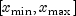is decomposed with a grid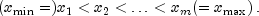On each subinterval the solution is represented by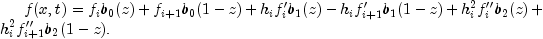The values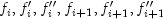are time-dependent coefficients associated with each interval. The basis functions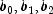are given for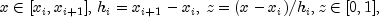by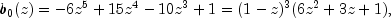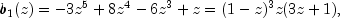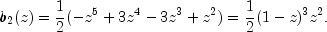By adding the piece-wise definitions the unknown solution function may be arranged as the series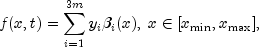where the time-dependent coefficients are defined by re-labeling: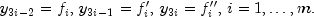The Galerkin principle is then applied. Using the provided initial and boundary conditions leads to an Index 1 differential-algebraic equation for the coefficients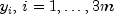.

This system is integrated using the variable order, variable step algorithm `DDASLX` noted in Hanson, R. and Krogh, F. (2008), Solving Constrained Differential-Algebraic Systems Using Projections. Solution values and their time derivatives at a grid preceding time T, expressed in units of time remaining, are given back by methods `getSplineCoefficients` and `getSplineCoefficientsPrime`, respectively. For further details of deriving and solving the system see Hanson, R. (2008), Integrating Feynman-Kac Equations Using Hermite Quintic Finite Elements. To evaluate f or its partials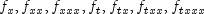at any time point in the grid, use method `getSplineValue`.

One useful application of the `FeynmanKac` class is financial analytics. This is illustrated in Example 2, which solves a diffusion model for call options which, in the special case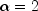reduces to the Black-Scholes (BS) model. Another useful application for the `FeynmanKac` class is the calculation of the Greeks, i.e. various derivatives of Feynman-Kac solutions applicable to, e.g., the pricing of options and related financial derivatives. In Example 5, the `FeynmanKac` class is used to calculate eleven of the Greeks for the same diffusion model introduced in Example 2 in the special BS case. These Greeks are also calculated using the BS closed form Greek equations (see http://en.wikipedia.org/wiki/The_Greeks). The Feynman-Kac and BS solutions are output and compared. Example 5 illustrates that the `FeynmanKac` class can be used to explore the Greeks for a much wider class of financial models than can BS.

Example 1, Example 2, Example 3, Example 4, Example 5, Serialized Form
• ### Nested Class Summary

Nested Classes
Modifier and Type Class and Description
`static interface ` `FeynmanKac.Boundaries`
Public interface for user supplied boundary coefficients and terminal condition the PDE must satisfy.
`static class ` `FeynmanKac.BoundaryInconsistentException`
The boundary conditions are inconsistent.
`static class ` `FeynmanKac.ConstraintsInconsistentException`
The constraints are inconsistent.
`static class ` `FeynmanKac.CorrectorConvergenceException`
Corrector failed to converge.
`static class ` `FeynmanKac.ErrorTestException`
Error test failure detected.
`static interface ` `FeynmanKac.ForcingTerm`
Public interface for non-zero forcing term in the Feynman-Kac equation.
`static class ` `FeynmanKac.InitialConstraintsException`
The constraints at the initial point are inconsistent.
`static interface ` `FeynmanKac.InitialData`
Public interface for adjustment of initial data or as an opportunity for output during the integration steps.
`static class ` `FeynmanKac.IterationMatrixSingularException`
Iteration matrix is singular.
`static interface ` `FeynmanKac.PdeCoefficients`
Public interface for user supplied PDE coefficients in the Feynman-Kac PDE.
`static class ` `FeynmanKac.TcurrentTstopInconsistentException`
The end value for the integration in time, tout, is not consistent with the current time value, t.
`static class ` `FeynmanKac.TEqualsToutException`
The current integration point in time and the end point are equal.
`static class ` `FeynmanKac.TimeIntervalTooSmallException`
Distance between starting time point and end point for the integration is too small.
`static class ` `FeynmanKac.ToleranceTooSmallException`
Tolerance is too small.
`static class ` `FeynmanKac.TooManyIterationsException`
Too many iterations required by the DAE solver.
• ### Field Summary

Fields
Modifier and Type Field and Description
`static int` `METHOD_OF_PETZOLD`
Used by method `setStepControlMethod` to indicate that the step control algorithm of the original Petzold code is used in the integration.
`static int` `METHOD_OF_SOEDERLIND`
Used by method `setStepControlMethod` to indicate that the step control method by Soederlind is used in the integration.
• ### Constructor Summary

Constructors
Constructor and Description
`FeynmanKac(FeynmanKac.PdeCoefficients pdeCoeffs)`
Constructs a PDE solver to solve the Feynman-Kac PDE.
• ### Method Summary

Methods
Modifier and Type Method and Description
`void` ```computeCoefficients(int numLeftBounds, int numRightBounds, FeynmanKac.Boundaries pdeBounds, double[] xGrid, double[] tGrid)```
Determines the coefficients of the Hermite quintic splines that represent an approximate solution for the Feynman-Kac PDE.
`double[]` `getAbsoluteErrorTolerances()`
Returns absolute error tolerances.
`int` `getGaussLegendreDegree()`
Returns the number of quadrature points used in the Gauss-Legendre quadrature formula.
`double` `getInitialStepsize()`
Returns the starting step size for the integration.
`int` `getMaximumBDFOrder()`
Returns the maximum order of the BDF formulas.
`double` `getMaximumStepsize()`
Returns the maximum internal step size used by the integrator.
`int` `getMaxSteps()`
Returns the maximum number of internal steps allowed.
`double[]` `getRelativeErrorTolerances()`
Returns relative error tolerances.
`double[][]` `getSplineCoefficients()`
Returns the coefficients of the Hermite quintic splines that represent an approximate solution of the Feynman-Kac PDE.
`double[][]` `getSplineCoefficientsPrime()`
Returns the first derivatives of the Hermite quintic spline coefficients that represent an approximate solution of the Feynman-Kac PDE.
`double[]` ```getSplineValue(double[] evaluationPoints, double[] coefficients, int ideriv)```
Evaluates for time value 0 or a time value in tGrid the derivative of the Hermite quintic spline interpolant at evaluation points within the range of `xGrid`.
`int` `getStepControlMethod()`
Returns the step control method used in the integration of the Feynman-Kac PDE.
`double` `getTimeBarrier()`
Returns the barrier set for integration in the time direction.
`void` `setAbsoluteErrorTolerances(double atol)`
Sets the absolute error tolerances.
`void` `setAbsoluteErrorTolerances(double[] atol)`
Sets the absolute error tolerances.
`void` `setForcingTerm(FeynmanKac.ForcingTerm forceTerm)`
Sets the user-supplied method that computes approximations to the forcing term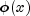and its derivative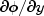used in the FeynmanKac PDE.
`void` `setGaussLegendreDegree(int degree)`
Sets the number of quadrature points used in the Gauss-Legendre quadrature formula.
`void` `setInitialData(FeynmanKac.InitialData initData)`
Sets the user-supplied method for adjustment of initial data or as an opportunity for output during the integration steps.
`void` `setInitialStepsize(double initStepsize)`
Sets the starting stepsize for the integration.
`void` `setMaximumBDFOrder(int maxBDFOrder)`
Sets the maximum order of the BDF formulas.
`void` `setMaximumStepsize(double maximumStepsize)`
Sets the maximum internal step size used by the integrator.
`void` `setMaxSteps(int maxSteps)`
Sets the maximum number of internal steps allowed.
`void` `setRelativeErrorTolerances(double rtol)`
Sets the relative error tolerances.
`void` `setRelativeErrorTolerances(double[] rtol)`
Sets the relative error tolerances.
`void` `setStepControlMethod(int stepControlMethod)`
Sets the step control method used in the integration of the Feynman-Kac PDE.
`void` `setTimeBarrier(double timeBarrier)`
Sets a barrier for the integration in the time direction.
`void` `setTimeDependence(boolean[] timeFlag)`
Sets the time dependence of the coefficients, boundary conditions and function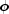in the Feynman Kac equation.
• ### Methods inherited from class java.lang.Object

`clone, equals, finalize, getClass, hashCode, notify, notifyAll, toString, wait, wait, wait`
• ### Field Detail

• #### METHOD_OF_PETZOLD

`public static final int METHOD_OF_PETZOLD`
Used by method `setStepControlMethod` to indicate that the step control algorithm of the original Petzold code is used in the integration.
Constant Field Values
• #### METHOD_OF_SOEDERLIND

`public static final int METHOD_OF_SOEDERLIND`
Used by method `setStepControlMethod` to indicate that the step control method by Soederlind is used in the integration.
Constant Field Values
• ### Constructor Detail

• #### FeynmanKac

`public FeynmanKac(FeynmanKac.PdeCoefficients pdeCoeffs)`
Constructs a PDE solver to solve the Feynman-Kac PDE.
Parameters:
`pdeCoeffs` - Implementation of interface PdeCoefficients that computes the values of the Feynman-Kac coefficients at a given point `(t,x)`.
• ### Method Detail

• #### computeCoefficients

```public void computeCoefficients(int numLeftBounds,
int numRightBounds,
FeynmanKac.Boundaries pdeBounds,
double[] xGrid,
double[] tGrid)
throws FeynmanKac.ToleranceTooSmallException,
FeynmanKac.TooManyIterationsException,
FeynmanKac.ErrorTestException,
FeynmanKac.CorrectorConvergenceException,
FeynmanKac.IterationMatrixSingularException,
FeynmanKac.TimeIntervalTooSmallException,
FeynmanKac.TcurrentTstopInconsistentException,
FeynmanKac.TEqualsToutException,
FeynmanKac.InitialConstraintsException,
FeynmanKac.ConstraintsInconsistentException,
SingularMatrixException,
FeynmanKac.BoundaryInconsistentException```
Determines the coefficients of the Hermite quintic splines that represent an approximate solution for the Feynman-Kac PDE.
Parameters:
`numLeftBounds` - an `int`, the number of left boundary conditions. It is required that
`1`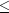`numLeftBounds``3`.
`numRightBounds` - an `int`, the number of right boundary conditions. It is required that
`1``numRightBounds``3`.
`pdeBounds` - Implementation of interface Boundaries that computes the boundary coefficients and terminal condition for given `(t,x)`.
`xGrid` - a `double` array containing the breakpoints for the Hermite quintic splines used in the `x` discretization. The length of `xGrid` must be at least 2, `xGrid.length >= 2`, and the elements in `xGrid` must be in strictly increasing order.
`tGrid` - a `double` array containing the set of time points (in time-remaining units) where an approximate solution is returned. The elements in array `tGrid` must be positive and in strictly increasing order.
Throws:
`FeynmanKac.ToleranceTooSmallException` - is thrown if the absolute or relative error tolerances used in the integrator are too small.
`FeynmanKac.TooManyIterationsException` - is thrown if the integrator needs too many iteration steps.
`FeynmanKac.ErrorTestException` - is thrown if the error test used in the integrator failed repeatedly.
`FeynmanKac.CorrectorConvergenceException` - is thrown if the corrector failed to converge repeatedly.
`FeynmanKac.IterationMatrixSingularException` - thrown if one of the iteration matrices used in the integrator is singular.
`FeynmanKac.TimeIntervalTooSmallException` - is thrown if the distance between an intermediate starting and end point for the integration is too small.
`FeynmanKac.TcurrentTstopInconsistentException` - is thrown if during the integration the current integration time and given stepsize is inconsistent with the endpoint of the integration.
`FeynmanKac.TEqualsToutException` - is thrown if during the integration process the actual integration time and the end time of the integration are identical.
`FeynmanKac.InitialConstraintsException` - is thrown if at the initial integration point some of the constraints are inconsistent.
`FeynmanKac.ConstraintsInconsistentException` - is thrown if during the integration process the constraints for the actual time point and given stepsize are inconsistent.
`SingularMatrixException` - is thrown if one of the matrices used outside the integrator is singular.
`FeynmanKac.BoundaryInconsistentException` - is thrown if the boundary conditions are inconsistent.
• #### getAbsoluteErrorTolerances

`public double[] getAbsoluteErrorTolerances()`
Returns absolute error tolerances.
Returns:
a `double` array of length `3*xGrid.length` containing absolute error tolerances for the solutions.
• #### getGaussLegendreDegree

`public int getGaussLegendreDegree()`
Returns the number of quadrature points used in the Gauss-Legendre quadrature formula.
Returns:
an `int`, the degree of the polynomial used in the Gauss-Legendre quadrature.
• #### getInitialStepsize

`public double getInitialStepsize()`
Returns the starting step size for the integration.
Returns:
a `double`, the starting step size used in the integrator.
• #### getMaximumBDFOrder

`public int getMaximumBDFOrder()`
Returns the maximum order of the BDF formulas.
Returns:
an `int`, the maximum order of the backward differentiation formulas used in the integrator.
• #### getMaximumStepsize

`public double getMaximumStepsize()`
Returns the maximum internal step size used by the integrator.
Returns:
a `double`, the maximum internal step size.
• #### getMaxSteps

`public int getMaxSteps()`
Returns the maximum number of internal steps allowed.
Returns:
an `int` specifying the maximum number of internal steps allowed between each output point of the integration.
• #### getRelativeErrorTolerances

`public double[] getRelativeErrorTolerances()`
Returns relative error tolerances.
Returns:
a `double` array of length `3*xGrid.length` containing relative error tolerances for the solutions.
• #### getSplineCoefficients

`public double[][] getSplineCoefficients()`
Returns the coefficients of the Hermite quintic splines that represent an approximate solution of the Feynman-Kac PDE.
Returns:
a `double` array of dimension `(tGrid.length+1) by (3*xGrid.length)` containing the coefficients of the Hermite quintic spline representation of the approximate solution for the Feynman-Kac PDE at time points `0, tGrid,...,tGrid[tGrid.length-1]`. Setting `ntGrid = tGrid.length` and `nxGrid = xGrid.length` the approximate solution is given by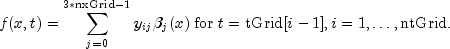The representation for the initial data at `t=0` is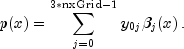The `(ntGrid+1) by (3*nxGrid)` matrix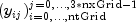is stored row-wise in the returned array.
• #### getSplineCoefficientsPrime

`public double[][] getSplineCoefficientsPrime()`
Returns the first derivatives of the Hermite quintic spline coefficients that represent an approximate solution of the Feynman-Kac PDE.
Returns:
a `double` array of dimension `(tGrid.length+1) by (3*xGrid.length)` containing the first derivatives (in time) of the coefficients of the Hermite quintic spline representation of the approximate solution for the Feynman-Kac PDE at time points `0, tGrid,...,tGrid[tGrid.length-1]`. The approximate solution itself is given by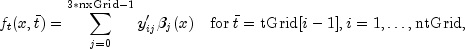and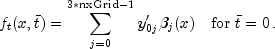The `(ntGrid+1) by (3*nxGrid)` matrix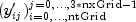is stored row-wise in the returned array.
• #### getSplineValue

```public double[] getSplineValue(double[] evaluationPoints,
double[] coefficients,
int ideriv)```
Evaluates for time value 0 or a time value in tGrid the derivative of the Hermite quintic spline interpolant at evaluation points within the range of `xGrid`.
Parameters:
`evaluationPoints` - a `double` array containing the points in `x-`direction at which the Hermite quintic spline representing the approximate solution to the Feynman-Kac PDE or one of its derivatives is to be evaluated. It is required that all elements in array `evaluationPoints` are greater than or equal to `xGrid` and less than or equal to `xGrid[xGrid.length-1]`.
`coefficients` - a `double` array of length `3*xGrid.length` containing the coefficients of the Hermite quintic spline representing the approximate solution f or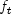to the Feynman-Kac PDE. These coefficients are the rows of the arrays `splineCoeffs` and `splineCoeffsPrime` returned by methods `getSplineCoefficients` and `getSplineCoefficientsPrime`. If the user wants to compute approximate solutions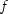or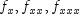to the Feynman-Kac PDE at time point `0`, one must assign row `splineCoeffs` to array `coefficients`. If the user wants to compute these approximate solutions for time points `t=tGrid[i], i=0,...,tGrid.length-1`, one must assign row `splineCoeffs[i+1]` to array `coefficients`. The same reasoning applies to the computation of approximate solutionsand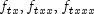and assignment of rows of array `getSplineCoefficientsPrime` to array `coefficients`.
`ideriv` - an `int` specifying the derivative to be computed. It must be 0, 1, 2 or 3.
Returns:
a `double` array containing the derivative of order `ideriv` of the Hermite quintic spline representing the approximate solution f orto the Feynman Kac PDE at `evaluationPoints`. If `ideriv=0`, then the spline values are returned. If `ideriv=1`, then the first derivative is returned, etc.
• #### getStepControlMethod

`public int getStepControlMethod()`
Returns the step control method used in the integration of the Feynman-Kac PDE.
Returns:
an `int` scalar specifying which step control method to be used.  Return Value Description `METHOD_OF_SOEDERLIND` Method of Soederlind `METHOD_OF_PETZOLD` Method from the original Petzold code DASSL
• #### getTimeBarrier

`public double getTimeBarrier()`
Returns the barrier set for integration in the time direction.
Returns:
a `double`, the time point beyond which the integrator should not integrate.
• #### setAbsoluteErrorTolerances

`public void setAbsoluteErrorTolerances(double atol)`
Sets the absolute error tolerances.
Parameters:
`atol` - a `double` scalar specifying the absolute error tolerances for the row-wise solutions returned by method `getSplineCoefficients`. The tolerance value `atol` is applied to all `3*xGrid.length` solution components. `atol` must be greater than or equal to zero. Also, not all entries in `atol` and `rtol` are allowed to be equal to 0 simultaneously. Default value is 1.0e-5 for each solution component.
• #### setAbsoluteErrorTolerances

`public void setAbsoluteErrorTolerances(double[] atol)`
Sets the absolute error tolerances.
Parameters:
`atol` - a `double` array of length `3*xGrid.length` specifying the absolute error tolerances for the row-wise solutions returned by method `getSplineCoefficients`. All entries in `atol` must be greater than or equal to zero. Also, not all entries in `atol` and `rtol` are allowed to be equal to 0 simultaneously. Default value is 1.0e-5 for each solution component.
• #### setForcingTerm

`public void setForcingTerm(FeynmanKac.ForcingTerm forceTerm)`
Sets the user-supplied method that computes approximations to the forcing termand its derivativeused in the FeynmanKac PDE.
Parameters:
`forceTerm` - a `ForcingTerm` interface specifying the user defined function used for computation of the forcing term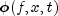and its derivative. If this member function is not called it is assumed thatis identically zero.
• #### setGaussLegendreDegree

`public void setGaussLegendreDegree(int degree)`
Sets the number of quadrature points used in the Gauss-Legendre quadrature formula.
Parameters:
`degree` - an `int`, the degree of the polynomial used in the Gauss-Legendre quadrature. It is required that `degree` is greater than or equal to 6. The default value is 6.
• #### setInitialData

`public void setInitialData(FeynmanKac.InitialData initData)`
Sets the user-supplied method for adjustment of initial data or as an opportunity for output during the integration steps.
Parameters:
`initData` - an `InitialData` object specifying the user-defined function for adjustment of initial data or the object can be used as an opportunity for output during the integration steps. If this member function is not called, no adjustment of initial data or output during the integration steps is done.
• #### setInitialStepsize

`public void setInitialStepsize(double initStepsize)`
Sets the starting stepsize for the integration.
Parameters:
`initStepsize` - a `double`, the starting stepsize used by the integrator. Must be less than zero since the integration is internally done from `t=0` to `t=tGrid[tGrid.length-1]` in a negative direction. The default is `initStepsize = -1.1102230246252e-16`.
• #### setMaximumBDFOrder

`public void setMaximumBDFOrder(int maxBDFOrder)`
Sets the maximum order of the BDF formulas.
Parameters:
`maxBDFOrder` - an `int` specifying the maximum order of the backward differentiation formulas used in the integrator. It is required that `maxBDFOrder` is greater than zero and smaller than 6. The default is `maxBDFOrder = 5`.
• #### setMaximumStepsize

`public void setMaximumStepsize(double maximumStepsize)`
Sets the maximum internal step size used by the integrator.
Parameters:
`maximumStepsize` - a positive scalar of type `double`, the maximum internal step size. Default value is `Double.MAX_VALUE`, the largest possible machine number.
• #### setMaxSteps

`public void setMaxSteps(int maxSteps)`
Sets the maximum number of internal steps allowed.
Parameters:
`maxSteps` - an `int` specifying the maximum number of internal steps allowed between each output point of the integration. `maxSteps` must be positive. The default value is 500000.
• #### setRelativeErrorTolerances

`public void setRelativeErrorTolerances(double rtol)`
Sets the relative error tolerances.
Parameters:
`rtol` - a `double` scalar specifying the relative error tolerances for the solution. The tolerance value `rtol` is applied to all `3*xGrid.length` solution components. `rtol` must be greater than or equal to zero. Also, not all entries in `atol` and `rtol` are allowed to be equal to 0 simultaneously. Default value is 1.0e-5 for each solution component.
• #### setRelativeErrorTolerances

`public void setRelativeErrorTolerances(double[] rtol)`
Sets the relative error tolerances.
Parameters:
`rtol` - a `double` array of length `3*xGrid.length` specifying the relative error tolerances for the solution. All entries in `rtol` must be greater than or equal to zero. Also, not all entries in `atol` and `rtol` are allowed to be equal to 0 simultaneously. Default value is 1.0e-5 for each solution component.
• #### setStepControlMethod

`public void setStepControlMethod(int stepControlMethod)`
Sets the step control method used in the integration of the Feynman-Kac PDE.
Parameters:
`stepControlMethod` - an `int` scalar specifying the step control method to be used in the integration. If this member function is not called `stepControlMethod` is set to `METHOD_OF_SOEDERLIND` by default.  `stepControlMethod` Description `METHOD_OF_SOEDERLIND` Use method of Soederlind. `METHOD_OF_PETZOLD` Use method from the original Petzold code DASSL.
• #### setTimeBarrier

`public void setTimeBarrier(double timeBarrier)`
Sets a barrier for the integration in the time direction.
Parameters:
`timeBarrier` - a `double`, controls whether the integrator should integrate in the time direction beyond a special point, `timeBarrier`, and then interpolate to get the Hermite quintic spline coefficients and its derivatives at the points in `tGrid`. It is required that `timeBarrier` be greater than or equal to `tGrid[tGrid.length-1]`. The default is `timeBarrier = tGrid[tGrid.length-1]`.
• #### setTimeDependence

`public void setTimeDependence(boolean[] timeFlag)`
Sets the time dependence of the coefficients, boundary conditions and functionin the Feynman Kac equation.
Parameters:
`timeFlag` - a `boolean` vector of length 7 indicating time dependencies in the Feynman-Kac PDE.  Index Time dependency of `0`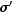`1``2`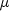`3``4` Left boundary conditions `5` Right boundary conditions `6``timeFlag[i] = true` indicates that the associated value is time-dependent, `timeFlag[i] = false` indicates that the associated value is not time-dependent. By default, `timeFlag[i] = false` for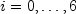.
JMSLTM Numerical Library 7.2.0